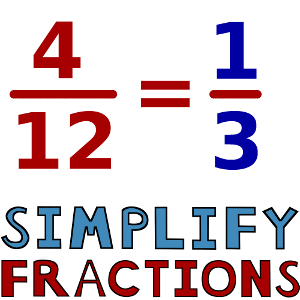Simplifying Fractions
3 years ago
lynchk
Save
Edit
Host a game
Live GameLive
Homework
Solo Practice
Practice17 QuestionsShow answers
• Question 1
30 secondsQ. Reduce 2/4
1/3
2/5
3/6
1/2
• Question 2
120 secondsQ. Simplify  9/18  to lowest terms.
1/2
3/6
9/18
5/10
• Question 3
120 secondsQ. Reduce 4/16  to lowest terms.
4/16
2/8
1/4
4
• Question 4
120 secondsQ. Simplify  70/80  to lowest terms.
35/40
7/8
9/10
14/16
• Question 5
120 secondsQ. Reduce 18/24  to lowest terms.
4/3
9/12
3/4
1/2
• Question 6
120 secondsQ. Simplify  5/40  to lowest terms.
2/20
5/40
8
1/8
• Question 7
120 secondsQ. Reduce 21/36  to lowest terms.
2/3
11/18
11/12
7/12
• Question 8
120 secondsQ. Simplify  6/9  to lowest terms.
2/3
1/5
3/4.5
1/3
• Question 9
120 secondsQ. Reduce 21/56  to lowest terms.
7/8
3/8
5/13
10/27
• Question 10
120 secondsQ. Simplify  9/36  to lowest terms.
4
3/12
1/4
12
• Question 11
30 seconds
Q. What number would you divide the numerator and denominator by when simplifying this fraction?  2/12
2
3
6
12
• Question 12
30 seconds
Q. What is the largest number you would divide the numerator and denominator by when simplifying this fraction?  4/16
2
4
8
16
• Question 13
30 seconds
Q. What number would you divide the numerator and denominator by when simplifying this fraction?  10/25
2
5
10
25
• Question 14
30 seconds
Q. What number would you divide the numerator and denominator by when simplifying this fraction?  6/9
2
3
6
9
• Question 15
30 seconds
Q. What number would you divide the numerator and denominator by when simplifying this fraction?  14/21
2
3
7
14
• Question 16
30 seconds
Q. What is the largest number you would divide the numerator and denominator by when simplifying this fraction?  20/50
2
5
10
20
• Question 17
30 seconds
Q. What number would you divide the numerator and denominator by when simplifying this fraction?  14/16